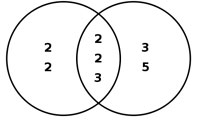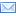#Difference Between Similar Terms and Objects

# Difference Between GCF and LCMGCF vs. LCM

The Greatest Common Factor (or the GCF) is the greatest real number shared between two integers. What makes this number a factor is that it is a whole, real number that two integers share –that is, when broken down to their lowest multiples, the largest integer that’s shared between the two numbers is their greatest common factor.

On the other hand, the Lowest Common Multiple (or LCM) is the integer shared by two numbers that can be divided by both numbers. Basically, in the list of two numbers’ respective list of multiples, the lowest number that the two numbers share is their lowest common multiple.

As regards the GCF, the greatest common factor must be a prime number –that is, a number that can only be divided by itself and 1. For example, the numbers 10 and 15 are broken down as such:

10: 1, 2, 5
15: 1, 3, 5, 15

When we take both sets of factors into consideration it is plain to see that the greatest prime integer shared by both numbers is 5 –it can only be divided by itself and 1 and it shows up in both 10 and 15.

However, as regards the LCM, the number must be composite (that is, it can be divided by at least itself, 1, and another multiple). Most likely the other multiple is shared between both numbers. For example, when creating a list of the multiples of 6 and 9:

6: 6, 12, 18, 24, 30…
9: 9, 18, 27, 36, 45…

As we can see, the lowest integer shared by both 6 and 9 is 18 –it is divisible by 1, 6, 9, and itself.

The biggest difference between the GCF and the LCM is that one is based upon what can divide evenly into two numbers (GCF), while the other depends on what number shared between two integers can be divided by the two integers (LCM). One must also consider if the numbers only share itself and 1 as common multiples of factors, than those numbers are not related to each other. That’s exactly what the GCF and LCM finds –how two whole numbers relate to each other.

Summary:
1. The GCF is based upon what integer divides evenly into two numbers; the LCM is based upon what integer two numbers share in a list of multiples.
2. The GCF must be a prime number; the LCM must be a composite number.

Latest posts by lancexpx (see all)

### Search DifferenceBetween.net :

Custom Search

Help us improve. Rate this post!(9 votes, average: 3.56 out of 5)Loading...Email This Post : If you like this article or our site. Please spread the word. Share it with your friends/family.

1. How do you compute the start-up costs for starting a website?

2. This article has a small error. The GCF does NOT have to be a prime number! For example the GCF of 64 and 56 is 8 and 8 is a composite number! Please fix the error

• Thank you you so much is is what iam was loking for …

3. Thanks for this it helped a lot

4. Thanks this really helped

5. One question… Is the GCF of 2 numbers greater than the LCM of the same numbers? Is this always true, never true, or sometimes true?

6. This is false! The GCF is NOT always prime.

For example 18 and 9 have share the greatest common factor of 9.

9 is NOT prime.

YIKES!

7. i still dont get it im new to this can you pls explain more of this especially for people who are new to learning this im not complaning its just hard for me to understand stuff thats new to me

• idk how to do this

Please note: comment moderation is enabled and may delay your comment. There is no need to resubmit your comment.

Articles on DifferenceBetween.net are general information, and are not intended to substitute for professional advice. The information is "AS IS", "WITH ALL FAULTS". User assumes all risk of use, damage, or injury. You agree that we have no liability for any damages.

See more about : , ,